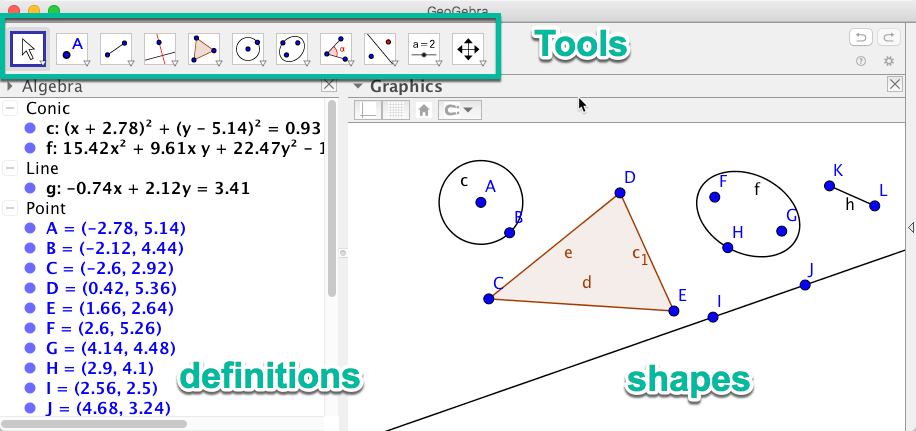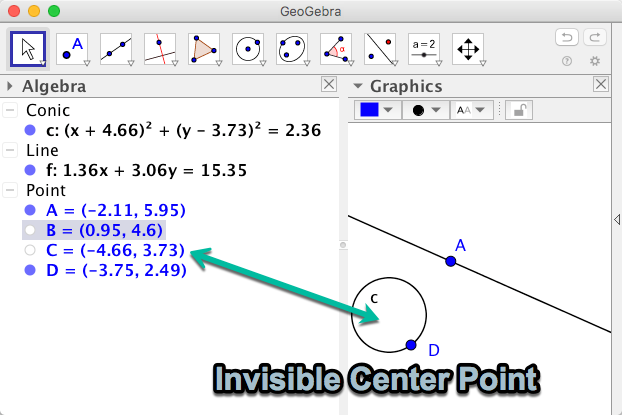# Software for Teaching Geometry

## GeoGebra is an amazing package, which replaces the use of a compass and ruler in teaching basics of geometry.

I have become a huge fan of a free multi platform application called GeoGebra 5, which offers an awesome way of learning and experimenting with different concepts in geometry.

The reason why I am writing about it is because I completely misunderstood the whole point of the application and how it works, for a long time. This made me not appreciate its genius.

I kind of treated it as a vector drawing program, because that is what I am used to. If you use it like that, then it is a cumbersome tool.

The best way to get the whole idea is to consider how would normally do geometry in school.

Do you remember creating a 45° angle like this using a compass and a ruler? This is exactly what GeoGebra lets you do but on the computer. Except it is far more flexible, as you can alter anything after you have drawn it.

You can hide an unhide different lines and points, or coloring lines in any color, to make it really easy to create very complex geometric shapes.

In GeoGebra making the same 45° angle, using the same method would end up looking something like this.

Which surely looks messy. This is because you draw full circles in GeoGebra, rather than partial ones’. However, since you can hide and show points and geometric shapes as you desire, it ends up being much easier to deal with complex constructions.

# How It Works

This is what GeoGebra would look like when I am normally using it.Main interface of GeoGebra 5. Shows most common tools and some common shapes drawin in the graphics area.

You got a bunch of different tools which allows you to decide whether you want to draw:

• points
• lines
• circles
• polygons

And many other geometric shapes. On the right you got the algebraic definitions of the points and shapes. You can use this to write precise positions for points e.g. or hide and unhide shapes. Here is an example. Say you got this circle and line and you want to hide point B and C.

Just click on the points in the definitions making them look hollow, like this:The center point of the circle has been made invisible as well as the second point B defining the line f.

## The Tools

Let’s just cover the most important tools for constructing geometry. Each button gives a drop down menu with related tools. Lets just cover the most important ones from left to right.

1. Move tool. I use this to select points, lines and shapes I want to change or move.
2. Point related tools. I use use this for adding point to lines and creating points from intersections between two lines.
3. Lines and line segments. Specifying two points gives you a line or a line segment.
4. Parallel and perpendicular lines.
5. Polygons. Usually I use this to draw lines created from intersections with lines and circles. E.g. you might inscribe a triangle inside a circle.
6. Circles. You can define circles in different ways, e.g. by a center and a radius or by two points.
7. Ellipses.
8. Angles. I use this to show the angles within triangles or intersecting lines I create.

So if you click on a tool button you get a drop down list like this.

The intersect tool is one I use frequently. It allows you to click on two intersecting lines to create a point at the intersection. This is needed when making lines, circles etc, as they require points to be defined.

Lets look at some common geometrical problems and how you would solve them.

# Perpendicular Line

A common problem is, given a line, how do you draw another line perpendicular to it? In GeoGebra there is already a tool to do this, but I want to show you how it would be done from scratch using a compass.

First you would use the line tool to define a line using two points marked in orange here.

Then you chose the tool circle with center through point, to draw a circle with the left most point as center, and the right most point defining the radius.

You repeat this, making a circle with the right most point as center, going through the left most point.

This will create two intersections. You create two points at these intersections using the intersect tool.

Now you can use the line tool again by clicking at each of the intersection points, to create a line going through them.

Create an intersection point at the center as well, since that will allow you to create an angle. Angles are made by selecting the angle tool and clicking 3 points.

## Changing the Geometry

Here is the whole point of GeoGebra. You can select the move tool and start moving the points defining the line, and you will see that your perpendicular line will move as well. Everything you have done thus far has created constraints which will be maintained as you move free points. You can’t move e.g. points from intersections as they are not free, but the result of constraints.

This allows you to demonstrate how various geometric relationships are retained even when you alter the size of circles, angles of lines etc.

Lets do one more example to show you how you solve geometrical problems with GeoGebra.

# Shortest Distance From a Point to a Line

Given a straight line and a point (in blue), and we want to find the shortest straight path to the line, how would we do it?

To create a line perpendicular to the one we have, we would need two points on that line. However we want to make sure this perpendicular line intersects our blue dot.

We know that a perpendicular line can be constructed in the middle of two points. Hence we need to create two points on the line which are at equal distance from the blue dot.

By drawing a circle which intersects the line, centered at the blue dot, we have guaranteed that both our intersection points in orange are at equal distance to the blue dot. They are both at the distance of the radius of the circle.

We can now create a perpendicular line using the orange intersection points in normal fashion. We do this by drawing two circles with a radius equal to the distance between the two orange points.

Drawing a line through the grey intersection points of the two circles gives us a perpendicular line running through the blue dot. This line is the shortest distance from the blue dot to our straight line.

Written by